# Poststack land data multiple removal example

In this section I attenuate in the poststack domain surface-related multiples with shaping filters that I estimate with theand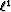norm. These filters are non-stationary. Figurea shows the multiple-infested data. Figureb displays the multiple model computed with a data-driven modeling approach Kelamis and Verschuur (2000). Note that for this gather, the amplitude differences between the primaries and the multiples are not very strong. My goal is to illustrate the use of thenorm in a more general case when surface-related multiples are present in the data. I specifically focus on the event at 1.6s in Figurea. This event is a primary that needs to be preserved by the subtraction procedure.

The amplitude of the primary at 1.6s is well preserved with thenorm in Figurea. Again,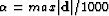gave a satisfying result. However, the amplitude of this primary is attenuated with thenorm as displayed in Figureb. Figureshows a comparison between the subtracted multiples with the(Figurea) and thenorm (Figureb). I conclude that thenorm tends to subtract too much energy.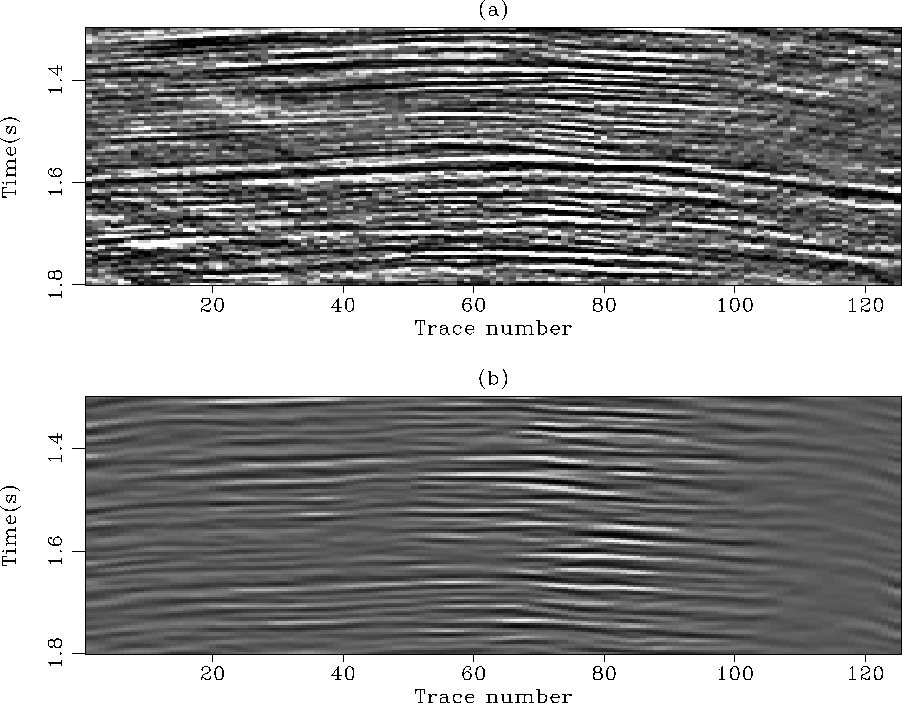win3
Figure 10
(a) Stack infested with multiples. (b) The multiple model computed with the Delft modeling approach. The subtraction is done poststack.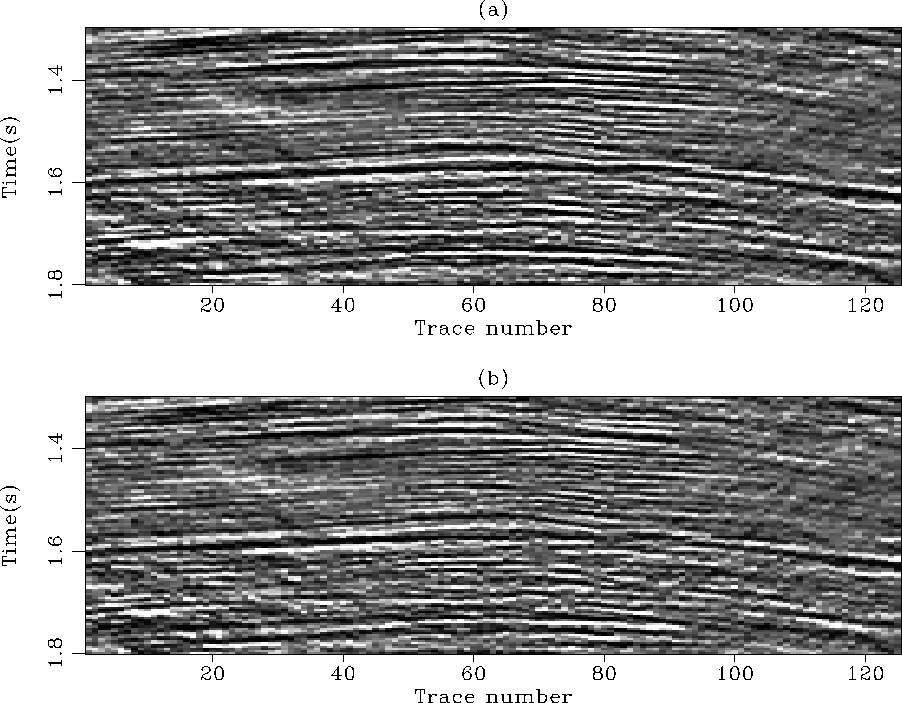win
Figure 11
(a) The estimated primaries withnorm adaptive subtraction. (b) The estimated primaries withnorm subtraction. The primary at 1.6s is very attenuated with thenorm. Thetechnique preserves its amplitude better.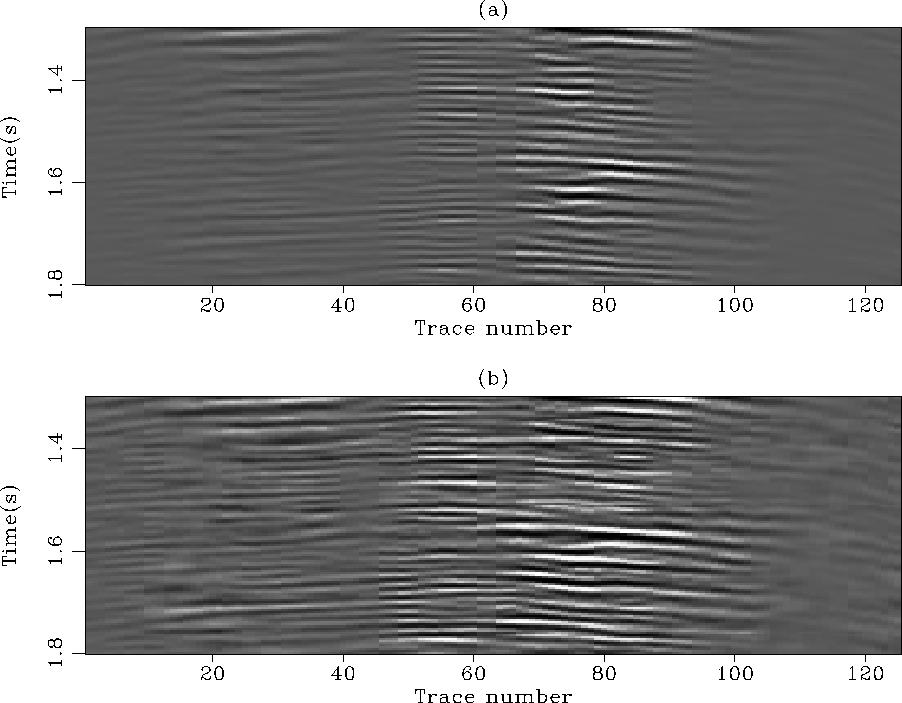win2
Figure 12
(a) The estimated multiples with thenorm subtraction. (b) The estimated multiples with thenorm subtraction. Thenorm tends to over-fit some multiples that creates some leaking of primaries in the estimated noise.This last example proves that the estimation of shaping filters can always be done with thenorm. An advantage of the inversion scheme with the Huber norm is that only one parameter (i.e.,) controls thebehavior. Thus it is easy to switch from one norm to another. Of course, it remains difficult to assert if the subtracted multiples with thenorm are more similar to the actual multiples than the subtracted multiples with thenorm. This judgment is only based on qualitative considerations for few known primary reflectors that need to be preserved.